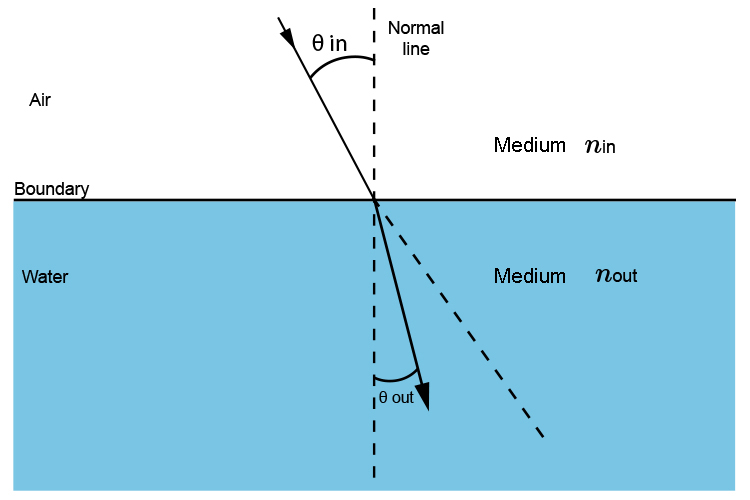# Snell's law is a ratio

Snell's law is a ratio and has no units.SNELL'S LAW we have learnt is

(sin\ \i\n\ \theta)/(sin\ \out\ \theta)=(n\ \(out))/(n\ \(i\n))

Which means it is a ratio of the angle going in against the angle going out

Ratio of (A\n\g\l\e\ \g\o\i\n\g\ \i\n)/(A\n\g\l\e\ \g\o\i\n\g\ \o\u\t)

Which is why N has no units.